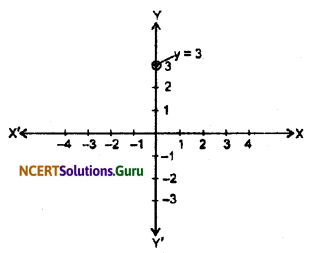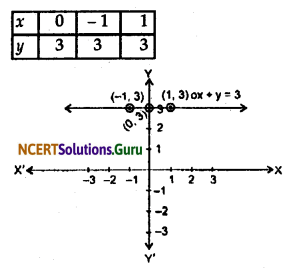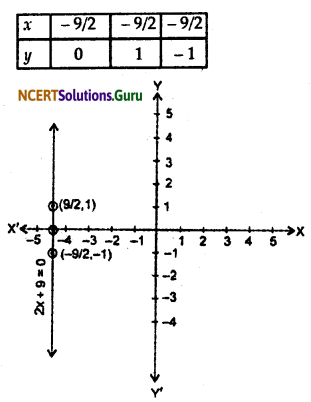# NCERT Solutions for Class 9 Maths Chapter 4 Linear Equations in Two Variables Ex 4.4

These NCERT Solutions for Class 9 Maths Chapter 4 Linear Equations in Two Variables Ex 4.4 Questions and Answers are prepared by our highly skilled subject experts.

## NCERT Solutions for Class 9 Maths Chapter 4 Linear Equations in Two Variables Exercise 4.4

Question 1.
Give the geometric representation of y = 3 as an equation.
(i) in one variable.
(ii) in two variables.
Solution:
(i) We have given that y = 3
It is treated as an equation in one variable y only.
It has a unique solution y = 3, which is a point on the graph.(ii) We have given that y = 3
It can be also expressed as
0 . x + y = 3
It is treated as an equation in two variables x and y.
It has infinitely many solutions which are in the form of (r, 3) where r is any real number.
The solutions of this equation represented by a line, we use the following table to draw the graphQuestion 2.
Give the geometric representations of 2x + 9 = 0 as an equation
(i) in one variable.
(ii) in two variables.
Solution:
(i) We have given that 2x + 9 = 0
or, x = $$\frac {-9}{2}$$
It is treated as an equation in one variable x only.
It has a unique solution x = $$\frac {-9}{2}$$, which is a point on the graph.(ii) We have given that 2x + 9 = 0
It can be also expressed as 2x + 0 . y + 9 = 0
It is treated as an equation in two variables x and y.
It has infinitely many solutions which are in the form of (r, 9) where r is any real number.
The solutions of this equation represented by a line.
To draw the graph we use the following table.error: Content is protected !!